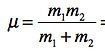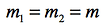Tutorial X:  The Diatomic Molecule: Nitrogen

Create a new folder for your work on this tutorial. Download the following files and put them in that folder:

PDB File: 10N2.pdb

FF Mod File:  N2.frcmod

INTRODUCTION:

A molecular mechanics model of any diatomic molecule consists of two point masses,      and     , connected by a covalent bond. The bond is modeled as a Hookean spring with ideal bond length     and force constant k.

The bond potential energy is

A diatomic molecule is an example of a compound harmonic oscillator. The period is

where     is the reduced mass,

For the nitrogen molecule,

and the last three equations can be combined to give the period: